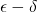# MA 425 content specifications

The content specifications are:

 A B C sequential characterization (2:1.4.2, 3:4, 5:2, 5:5, 5:3.4.6) formulate and prove prove at least 2 prove at least 1 Cauchy criteria formulate and prove prove at least 1 (5:3.2.5, 4) use 1 Archimedean Principles prove all are equivalent (2:6 and 2:8 together) use each of AP1, AP2, AP3 forms of completeness use Nested Intervals prove 2 are equivalent (2:5,4:2, 4:4) compactness use open-covers version use Heine-Borel version use sequential compactness limits and continuity use topological version of continuity to show a function is continuous prove two definitions of continuity are equivalent use theversion of continuity to show a function is continuous use the sequential criterion for continuity to show a function is continuous major/named theorems prove 2 at infinity" versions of named theorems (7:8, 8:3, 9: 1-6) prove an at infinity" version of a named theorem (7:8, 8: 3, 9: 1-6) prove at least one (6:11, 7:5) derivatives use Taylor's Theorem use Caratheodory's Criterion use Mean Value Theorem use the limit definition of the derivative integration compute 3 integrals with proof compute 2 integrals with proof compute 1 integral with proof convergence of functions prove a sequence converges pointwise but not uniformly series prove series versions of 2 named theorems for sequences prove series version of a named theorem for sequences explanation points 3 points 2 points 1 point pathologies & exotica points 3 points 2 points 1 point topology points 3 points 2 points 1 point

• Numbers in italics represent problems that definitely satisfy that specification. 3:4 means problem 4 from homework 3. 2:1.4.8 means Abbott's exercise 8 from chapter 1.4, which was assigned on homework 2.
• formulate and prove means you must come up with the statement of the theorem, in addition to proving it.
• use means to use the result in question in the course of a proof
• a named theorem is one with a person's name attached (such as the Bolzano-Weierstrass Theorem) or a phrase (such as the Mean Value Theorem)
• a sequential characterization or a sequential criterion is a theorem of the form [statement not involving sequences] if and only if [statement involving sequences]. Examples include:
• The sequential characterization of supremum.
• The sequential criterion for continuity.
• The sequential characterization of closed sets.
• forms of completeness are: the Monotone Sequence Property, the Least Upper Bound Property, the Greatest Lower Bound Property, and the Bolzano-Weierstrass Property

Points can be earned by completing the following problems. Topology points may also be earned by using arguments that reference open, closed, and/or compact sets. Explanation points may also be earned by giving a very clear unsolicited explanation as part of one of your proofs.

 explanation 2:2, 3:2, 8:4, 10:9 pathologies & exotica 1:3, 2: 1.4.8, 6: 6, 3.3.7 9: 7b 10: 7.3.9(d), 8 topology 7:2 10:10